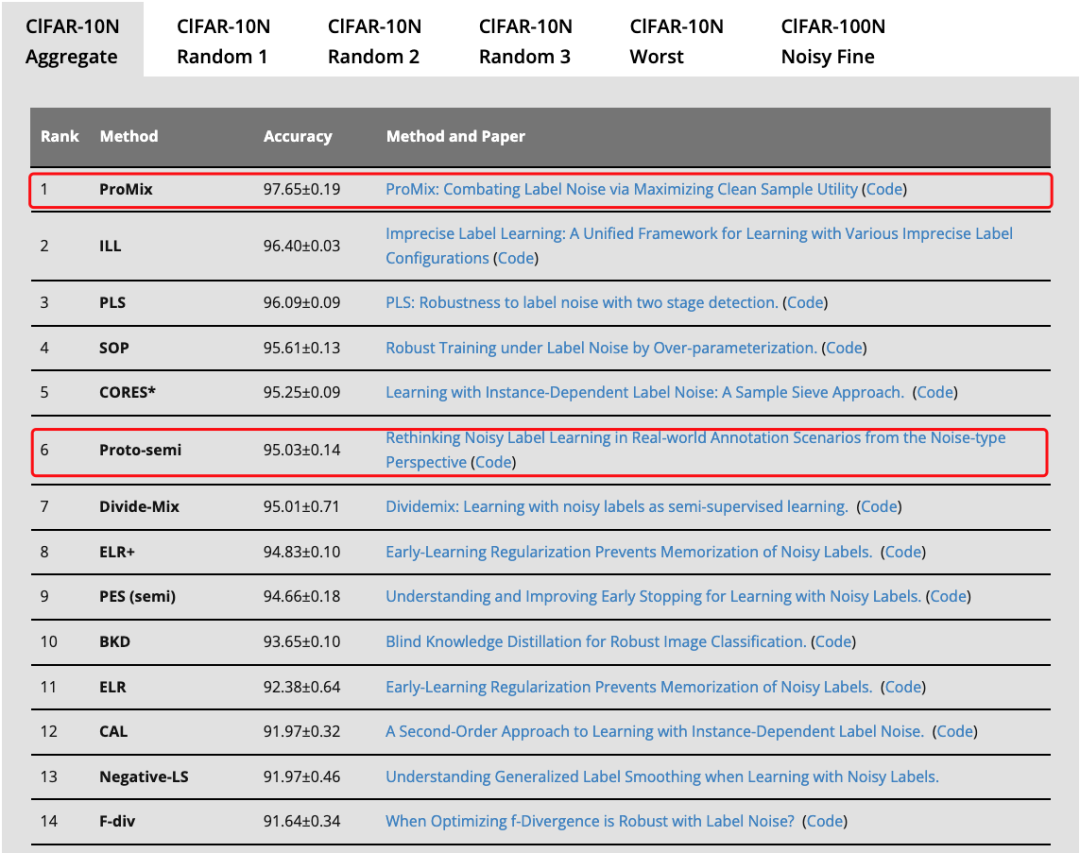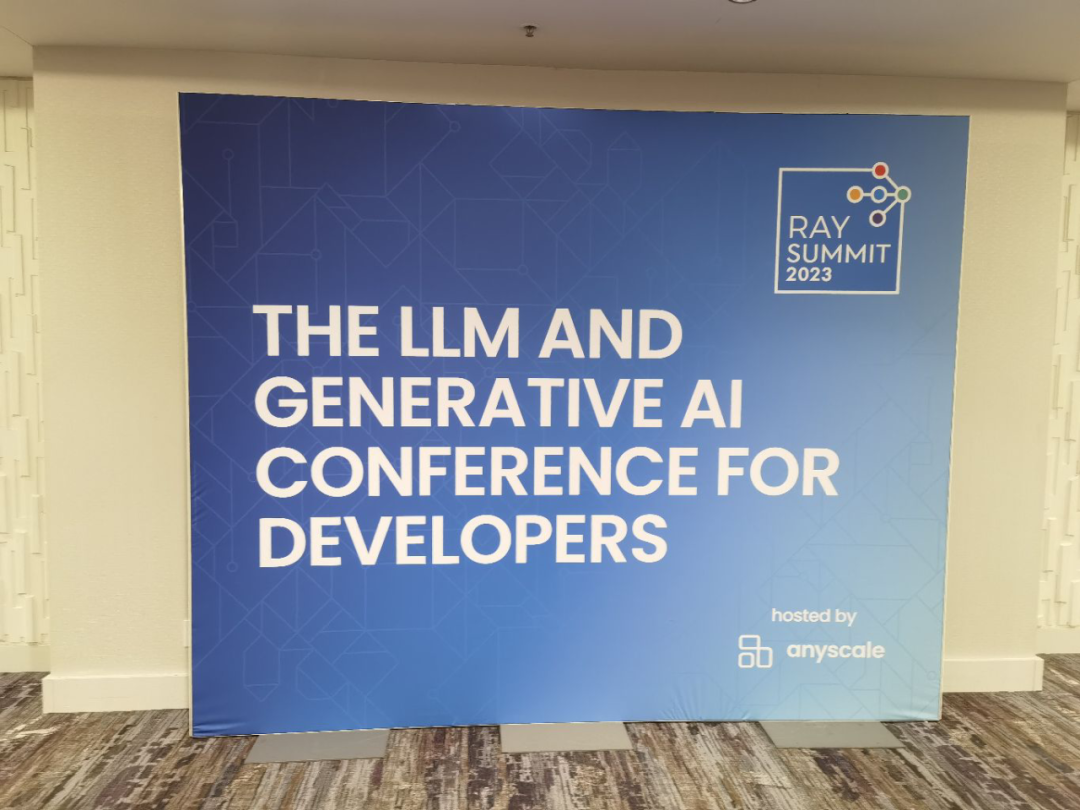# 使用TensorFlow和Keras构建深度学习模型

TensorFlow和Keras是当今最受欢迎的深度学习框架之一。不仅提供了高级API，使得深度学习模型的构建和训练变得非常容易，还提供了多种层和模型类型，可以轻松地构建各种类型的深度学习模型。因此被广泛用于训练大规模的深度学习模型。

``import tensorflow as tffrom tensorflow import kerasfrom tensorflow.keras import layersfrom tensorflow.keras.datasets import cifar10# 加载CIFAR-10数据集(x_train, y_train), (x_test, y_test) = cifar10.load_data()# 将像素值缩放到0到1之间x_train = x_train.astype("float32") / 255.0x_test = x_test.astype("float32") / 255.0# 将标签从整数转换为one-hot编码y_train = keras.utils.to_categorical(y_train, 10)y_test = keras.utils.to_categorical(y_test, 10)``

``model = keras.Sequential(    [        # 第一个卷积层        layers.Conv2D(32, (3, 3), activation="relu", input_shape=(32, 32, 3)),        layers.MaxPooling2D((2, 2)),        # 第二个卷积层        layers.Conv2D(64, (3, 3), activation="relu"),        layers.MaxPooling2D((2, 2)),        # 第三个卷积层        layers.Conv2D(128, (3, 3), activation="relu"),        layers.MaxPooling2D((2, 2)),        # 展平层        layers.Flatten(),        # 全连接层       layers.Dense(128, activation="relu"),        layers.Dense(10, activation="softmax"),    ])``

``# 编译模型model.compile(optimizer="adam", loss="categorical_crossentropy", metrics=["accuracy"])# 训练模型history = model.fit(x_train, y_train, epochs=10, validation_data=(x_test, y_test))``

``# 在测试集上评估模型test_loss, test_acc = model.evaluate(x_test, y_test)print("Test loss:", test_loss)print("Test accuracy:", test_acc)``

``import matplotlib.pyplot as plt# 绘制训练和验证损失plt.plot(history.history["loss"], label="Training loss")plt.plot(history.history["val_loss"], label="Validation loss")plt.xlabel("Epoch")plt.ylabel("Loss")plt.legend()plt.show()# 绘制训练和验证准确率plt.plot(history.history["accuracy"], label="Training accuracy")plt.plot(history.history["val_accuracy"], label="Validation accuracy")plt.xlabel("Epoch")plt.ylabel("Accuracy")plt.legend()plt.show()``

2023-09-27 10:07:19

2023-09-27 10:02:13

2023-09-26 09:57:22CycleGAN（基于深度学习的图像转换模型）
CycleGAN是一种基于深度学习的图像转换模型，它可以将一种类型的图像转换成另一种类型的图像，例如将马的图像转换成斑马的图像，将夏季景色的图像转换成冬季景色的图像等等。这种图像转换技术具有广泛的应用前景，例如在计算机视觉、虚拟现实、游戏开发、图像增强等领域。
2023-09-22 10:12:16

2023-09-22 09:59:122023-09-21 09:55:18

Attention机制是一种处理序列数据的重要算法，其核心思想是为每个输入序列中的元素分配一个权重，以便在计算输出时考虑这些元素的相对重要性。这种机制已经被广泛应用于自然语言处理、图像处理以及其他领域。下面将介绍几种基于attention机制的算法及其应用。
2023-09-20 10:08:33
Attention模型详解
Attention模型是深度学习中的一种重要模型，它能够帮助模型处理序列数据，从而在机器翻译、语音识别、图像处理等领域中取得了很好的效果。本文将详细介绍Attention模型的原理、应用和发展。
2023-09-20 10:04:47
svm网格搜索参数寻优过程
SVM是一种经典的监督学习算法，常用于分类和回归问题。SVM的核心思想是找到一个最佳的超平面，将不同类别的数据分隔开来。SVM网格搜索是一种常用的参数优化方法，通过对不同的参数组合进行试验，寻找最优的参数组合，以提高模型的性能。
2023-09-15 10:27:02

Bert是一种基于Transformer架构的预训练语言模型，可以用于各种自然语言处理任务，包括文本分类。在本文中，我们将详细介绍如何使用Bert预训练模型进行文本分类。
2023-09-15 10:07:23